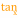# Tangent

 Block Group: Trigonometric Functions Icon:The Tangent block returns the tangent of the given angle.

For information on using dataflow blocks, see Dataflow.

## Input/Output Property

The following property of the Tangent block can take input and give output.

• input (number)

input specifies the angle to get the tangent for, in degrees.

## Output Property

The following property of the Tangent block can give output but cannot take input.

• output (number)

output returns the tangent of the input.

## Example

The following image shows an example of the Tangent block. In this example, the angle is 40, and the tangent is 0.839.